# Fibre Bundles

John Robinson has explained in discussing ETERNITY how he was fascinated by the Möbius Band, and the way it can be seen as a line segment twisting as it moves around a circle. Instead of a line segment, different shapes can be moved round a circle, and with a twist of various amounts. These shapes may match up, but it depends on the shape you start with, and the amount of twist. In this way John discovered a general concept which is very important in mathematics, that of a fibre bundle.

The structure of a fibre bundle consists of a base, which for the Möbius Band and other of John's sculptures, is a circle. The fibre is the form of the shape which is moved around the circle. The extra data to describe the object is the amount of twist in the fibre as it moves round the circle. This amount of twist has to be related to the shape of the fibre, so that the fibres match. For a Möbius Band, the fibre is just a line segment and the twist is 180 degrees.

 For a fibre with three fold symmetry, such as a triangle, the twist could be any whole number multiple, including zero, of 120 degrees. A twist of exactly 120 degrees gives John's sculpture ETERNITY. This sculpture can be seen in the steps of construction below, which shows clearly the triangular fibre of the fibre bundle, and the way the fibres fit together.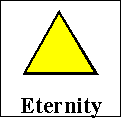### The making of Eternity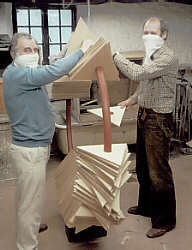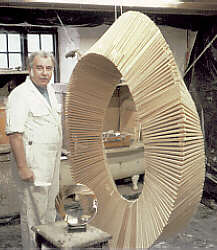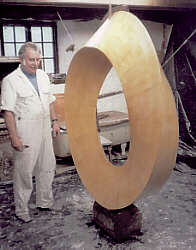Roy Wakeford, Master Plasterer

1. Helping to place the triangles on the central circle
2. Fixing the triangles in place with the added twist
3. With the finished plaster ready for the foundry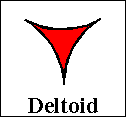If the triangular fibre in ETERNITY is replaced by a deltoid then the resulting form occurs in singularity theory and is known as the umbilic bracelet *. The USA artist and mathematician Helaman Ferguson has used symbolic computation to control the making of an exact sculpture of this form.

 If the fibre is taken to be three touching circles, and the twist is eight times 120 degrees, then we obtain the GORDIAN KNOT. There is also an animation of this.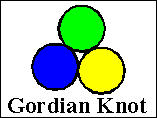For a square, or for any object with four fold symmetry, the twist could be any multiple of 90 degrees. A twist of 180 degrees yields John's sculpture DEPENDENT BEINGS. In order to capture John's colouring of the faces of this, we have made a rotating model from two bands, each of which is a fibre bundle over a circle with fibre a line segment and a twist of 360 degrees.

What John has shown is that these forms which can be described in exact mathematical terms can also, with the right choice of proportions and with exact craftmanship, be made into objects of astonishing beauty. The reasons for the choice of these proportions are not at present part of the subject of mathematics.

Thus there are areas into which mathematics does not at present venture.

But there are also areas into which it seems difficult for art to venture, but into which mathematics does. The notion of a fibre bundle is so general that it describes new kinds of objects which are difficult to visualise as they may be realised properly only in a space of many dimensions, or have to be constructed as a concatenation of given forms and data. Suppose for example that we replace the circular base of the Möbius Band by a more complex object such as the surface of a sphere. The fibre could also be, not a triangle or square, but one of the five Platonic solids, for example a tetrahedron or a cube. How can we describe and understand the geometry, the spatial interelations, of the objects which result? How many different kinds of such objects are there? This kind of curiosity is one of the motivating factors of mathematics. We also see the standard problem types of representation and classification. Such problems are seen again in the study of knots, as shown in the Knot Exhibition.

Such curiosity is also not idle. Objects of this kind arise in descibing many physical situations and in many geometrical areas. The tools which mathematicians have developed over many decades to understand and calculate with these strange objects have been found to be useful in a range of other parts of mathematics and its applications.

In a further page, Classification, we discuss the deeper problem of classifying in various ways objects that may be constructed as fibre bundles.

*...`Geometric Differentiation for the intelligence of curves and surfaces', by I.R.Porteous, Cambridge University Press 1994, page 134.© Mathematics and Knots/Edition Limitee 1996 - 2002
This material may be used freely for educational, artistic and scientific purposes, with acknowledgement, but may not be used for commercial purposes, for profit or in texts without the permission of the publishers.